## Wednesday, 1 February 2012

### Generating functions part 3: constant from integration

This passage surrounds the following problem.

Find the general formula for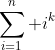where k can be any non-negative number.

We know that a sum of polynomial of degree n would give polynomial of degree (n+1) by knowledge similar to integration, so we are not going to find the general formula through k, but through n for any fixed k.

For the first few k many of you should have already recited these:
1)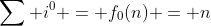, this is the series 1+1+1+...
2), this is the summation of A.S..
3)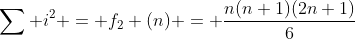4)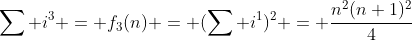For fixed k we only need to differentiate/integrate w.r.t. n, it seems easy: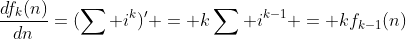.
Yes this is a diff. w.r.t. n (the summation formula is in terms of n, so we differentiate w.r.t. i in the summation sign.), but it looks like it's differentiate w.r.t k.

However, this would be a good news to us because we can link functions of different k together, while smaller k is trivially to compute.

We try to differentiate a few of them:
1)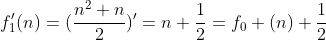2)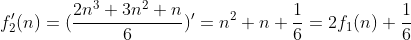3)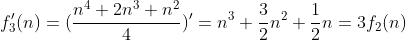Noticing the varying constant at the last, what happened?

Constant remind us about the constant results from integration, but why we get such a constant from integration, and are there any formula to compute that constant?

In elemental approach we can explain it like this by integration: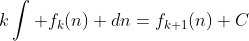Now note that this C depends of the initial value of f(n), now we know that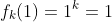, so C is equal to zero because the function does not contain any other constant other than the integration concerning the polynomial.

However, the constant of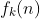is not necessarily related to the constant of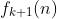, then we have to determine the constant again. For constant with non-zero degree, i.e.,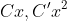, it's no longer vary along to initial value, so it could be fixed for further integration.

Now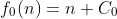where C=0 for k=0.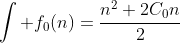, by putting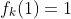we have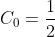However, it's extremely hard to generate the general formula of the constant because it's in a special recurrence form.

Let's try the formula for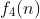: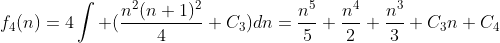, putting n=1 gives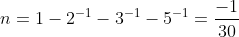.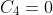for k=4, though it will vary for larger k. Now we get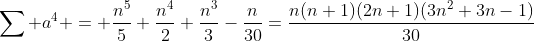. Try the first few term which is correct.

Problem to explore:
1) Show thatand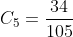, hence find the formula for k=5,6,7 and try the first few terms.
2) Prove or disprove that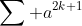is divisible by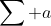for all natrual n,k.